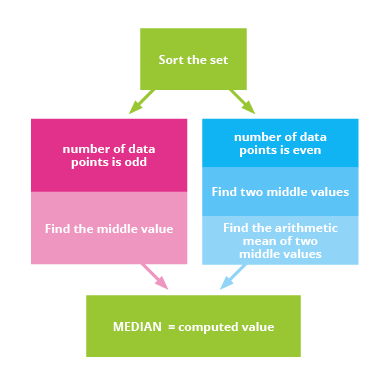Deals Of The Week - hours only!Up to 80% off on all courses and bundles.-Close
Introduction
Arithmetic mean
Median
7. Median – construction procedure
Mode
Summary

## Instruction

The first step in calculating the median is to sort the set elements into sequential order. Next, depending on the parity of the set (whether there is an odd or even number of elements), you have to find the one or two middle values. As we mentioned before, if there is an odd number of elements, the result is the middle observation. If there is an even number of elements, the answer is the arithmetic mean of the two middle observations.For instance, consider the set:

0, 1, 2, 3, 4, 5, 1, 2, 3, 1, 2, 3

After ordering, we get:

0, 1, 1, 1, 2, 2, 2, 3, 3, 3, 4, 5

The set has an even number of elements, so we look for the two middle values, which in this case are 2 and 2. Calculating the arithmetic mean, we get 2as the resulting median.